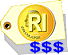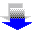RICalcFinancialInvestmentInflationGraphingStatisticsScientific / Other DownloadsFree demo Online shopBuy online AboutContact us LinksResources

 Take a Tour For Home For ProfessionalsBrochure
(328 KB)# Graphing calculator

Graphing calculator for solving and graphing mathematical equations.

RICalc software for Algebra, Calculus, Trigonometry and general mathematics.

Solve and graph equations in 2D rectangular, parametric and polar coordinates.

Graph algebraic equations or define matrix points and graph smooth from low to high quality.

View report of Roots, Extrema, Intercept, Distance and Table points.All calculators in one
Foreign Exchange indicative price

Graphing
, Scientific, Statistics, Inflation, Financial, Investment

## How can you use the Graphic calculator?

Find solutions of algebraic equations, calculate integrals and derivatives and more..

Ever wonder what is the shape of an equation? Just graph it and trace it.Maths equation graphing

Calculus featuresSolve equations with choice of multiple root finding methods or find minimum and maximum.Calculate Derivatives with multiple evaluation methods.Calculate Integrals with multiple evaluation methods.

Work with predefine functions for Maths, Trigonometry, Statistic, Financial, Conversion, Logical, Text, Date, Information, Geometry and other functions, or create own expressions.

Graphing features
Plot Tangent or Normal at a point, plot Distance between points, plot Derivative.

Trace expression on the plane with mouse or use keyboard for fine steps.

Zoom all or individual X and Y, move up, down, left, right, centre and restore to original.

Control plot Steps and Interval, Limits, Points or Lines, Maximum and Minimums, style, width, colour ...® Product and company names are trademarks or registered trademarksPrivacyDisclaimerSite Map © 2020 DBCOM software